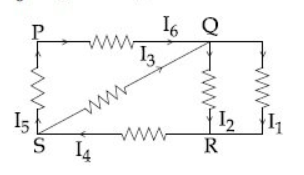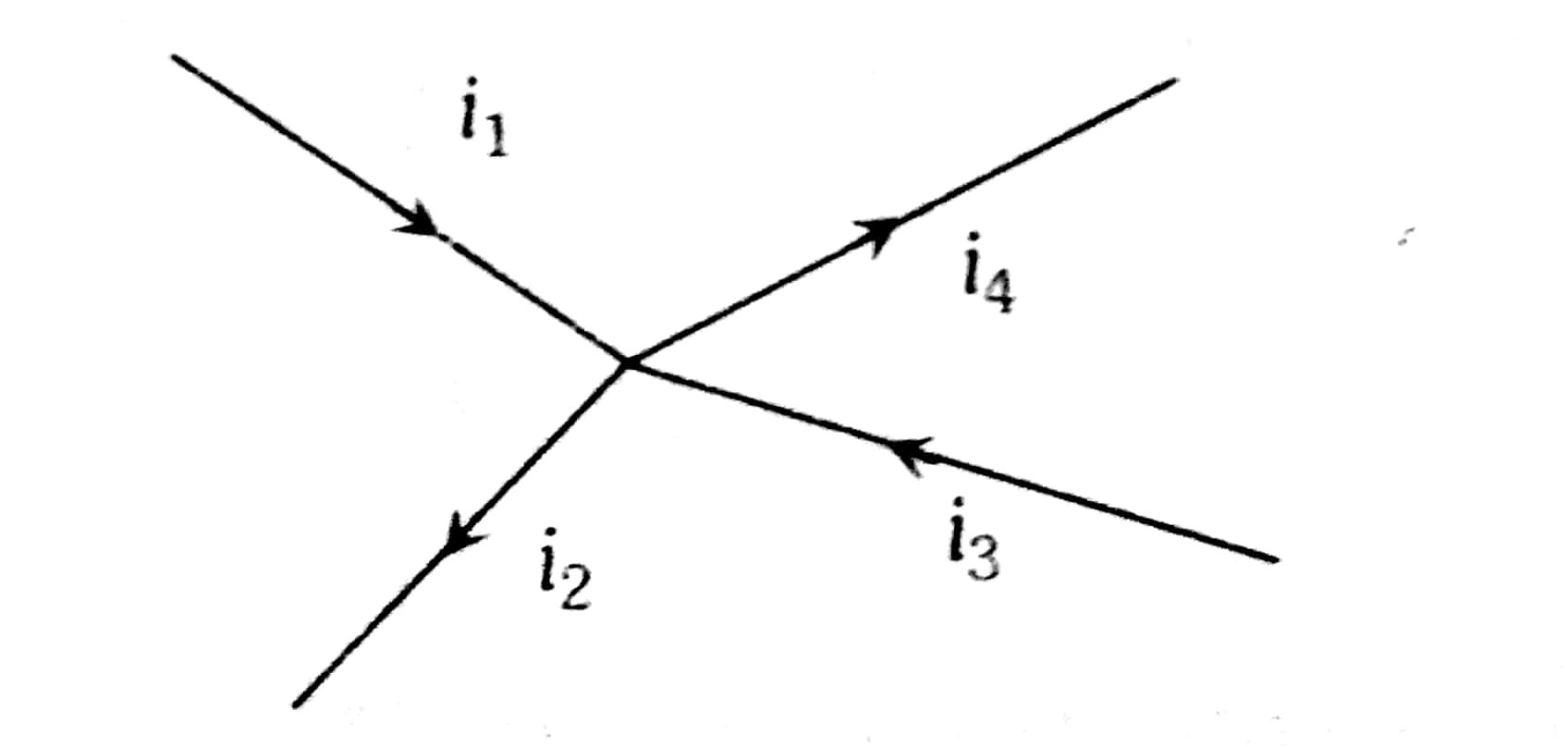Q

# Try this! In the given circuit diagram, the currents, I1= -0.3 A,I4 = 0.8A and I5 = 0.4A, areflowing as shown. The currents I2, I3 and I6,respectively, are :

In the given circuit diagram, the currents, I1 = -0.3 A, I4 = 0.8 A and I5 = 0.4 A, areflowing as shown. The currents  I2 , I3  and  I6 ,respectively, are :• Option 1)

-0.4 A, 0.4 A, 1.1 A

• Option 2)

1.1 A -0.4 A, 0.4 A

• Option 3)

0.4 A,1.1 A ,0.4 A

• Option 4)

1.1 A ,0.4 A,0.4 A

Views

Kirchoff's first law -

- whereinApply Kcl at Q

I6+I3 = I1+ I2   .........(1)

I1 = 0.3A

I4 = 0.8A

I5 = 0.4A

KCL at 5

I4= I5 +I3

I3 = I4 - I5

= 0.8 +0.4

=0.4A

KCL at R

I1+I2 = I4

I2 = I4 - I1

= 0.8 - (-0.3)

=1.1A

From equation (1)

I6 + I3 = I1+ I2

I6 = I1 + I2 - I3

= -0.3+1.1 -0.4

=0.4A

I2 = 1.1A

I3 = 0.4A

I6 = 0.4A

Option 1)

-0.4 A, 0.4 A, 1.1 A

Option 2)

1.1 A -0.4 A, 0.4 A

Option 3)

0.4 A,1.1 A ,0.4 A

Option 4)

1.1 A ,0.4 A,0.4 A

Exams
Articles
Questions